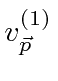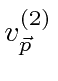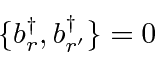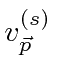## The Quantized Dirac Field with Positron Spinors

The basis states in our quantized Dirac field can be changed eliminate the negative energy'' states and replace them with positron states. Recall that we can replacewith the positron spinorandwithsuch that the new spinors are charge conjugates of the electron spinors.The positron spinor is actually just the same as the negative energy spinor when the momentum is reversed.

We name the creation and annihilation operators for the positron states to beandand identify them to be.These anti-commute with everything else with the exception thatThe Dirac field and Hamiltonian can now be rewritten.All the energies of these states are positive.

There is an (infinite) constant energy, similar but of opposite sign to the one for the quantized EM field, which we must add to make the vacuum state have zero energy. Note that, had we used commuting operators (Bose-Einstein) instead of anti-commuting, there would have been no lowest energy ground state so this Energy subtraction would not have been possible. Fermi-Dirac statistics are required for particles satisfying the Dirac equation.

Since the operators creating fermion states anti-commute, fermion states must be antisymmetric under interchange. Assumeandare the creation and annihilation operators for fermions and that they anti-commute.The states are then antisymmetric under interchange of pairs of fermions.Its not hard to show that the occupation number for fermion states is either zero or one.

Note that the spinors satisfy the following equations.Since we changed the sign of the momentum in our definition of, the momentum term in the Dirac equation had to change sign.

Jim Branson 2013-04-22